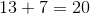# ISEE Middle Level Math : How to find the square root

## Example Questions

### Example Question #31 : Squares / Square Roots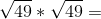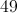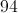Explanation:

First, find the sqaure root :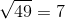Then solve accordingly: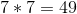### Example Question #32 : Squares / Square Roots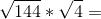Explanation:

FInd the square roots: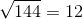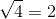Then, solve: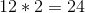### Example Question #33 : Squares / Square Roots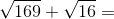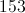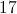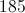Explanation:

First, find the square roots: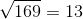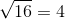Then, solve accordingly: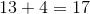### Example Question #34 : Squares / Square Roots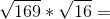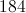Explanation:

First, find the square roots:Then, solve:### Example Question #35 : Squares / Square Roots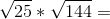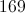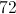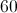Explanation:

First find the square roots: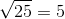Then, solve: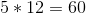### Example Question #36 : Squares / Square Roots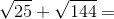Explanation:

First find the square roots:Then, solve: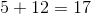### Example Question #37 : Squares / Square Roots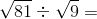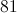Explanation:

First find the square roots: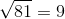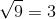Then, solve: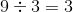### Example Question #38 : Squares / Square Roots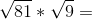Explanation:

First, find the square roots:Then, solve: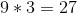### Example Question #39 : Squares / Square Roots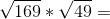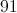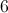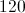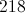Explanation:

First, find the sqaure roots:Then, solve: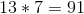### Example Question #40 : Squares / Square Roots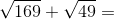Explanation:

First, find the sqaure roots:Then, solve: